﻿ 人字形支护结构内力与变形分析及应用

人字形支护结构内力与变形分析及应用Analysis and Application of Internal Force and Deformation of Herringbone Bracing Structure

Abstract: On the basis of summarizing, analyzing and sorting out the characteristics of double-row pile supporting structure, the subject group puts forward the herringbone supporting structure, which is a new form of deep foundation pit supporting structure. In this paper, based on the ba-sic idea of Winkler foundation beam, the vertical friction effect between row piles and soil and the space effect are neglected, and the friction effect between inclined reinforced cement-soil Wall and soil is considered. The plane finite element model of herringbone bracing structure considering pile-soil effect is established, and the global stiffness Matrix of herringbone bracing structure is constructed, and the corresponding Matlab Program is compiled. The analysis of in-ternal force and deformation is carried out through an engineering case. The results show that the calculated displacement is consistent with the measured displacement, and the internal force is regular and comparable, which can be used as a reference for the design of herringbone bracing structure.

1. 引言

2. 人字形支护结构的构造及优点

1) 稳定性好。斜向加筋水泥土地下连续墙锚体与钢筋混凝土排桩形成空间支护结构，增强了墙后土体稳定性，能很好控制基坑变形。

2) 支护能力强。斜向加筋水泥土地下连续墙锚体的抗拔力由地下连续墙与土的摩擦阻力和地下连续墙自重组成。由于地下连续墙自重大，且其侧面与土接触的面积大，摩擦阻力大，因此，斜向加筋水泥土地下连续墙锚体能提供比普通锚杆高很多的抗拔力。

3) 集挡土、止水与锚固、托换与隔离功能于一体。斜向加筋水泥土地下连续墙既有挡土功能，又有止水功能，还兼有锚杆功能，同时，对邻近基坑的坡顶建(构)筑物还有托换及隔离作用。

4) 控制支护结构变形及周边地面沉降能力强。因斜向加筋水泥土地下连续墙的斜向支承及遮拦作用，致使作用在钢筋混凝土排桩上的土压力降低，减少了支护结构的变形及桩身内力，并且还可以通过对加筋体施加合适的预应力来控制支护结构的变形及地面沉降。

5) 坑底抗隆起稳定性强。因斜向加筋水泥土地下连续墙的存在，一方面提高了可能发生的滑动面上的抗剪能力；另一方面从整体上提高了坑外土体的压缩模量等土性指标，起到了加固坑外土体的作用，从而提高了基坑的抗隆起稳定性。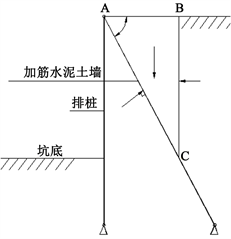Figure 1. Simplified diagram for calculation of earth pressure

3. 人字形支护结构平面有限元模型

3.1. 基本假定

1) 忽略排桩与土的竖向摩擦效应及空间效应，假设人字形支护结构为平面杆件体系；

2) 假设排桩桩底及斜向加筋水泥土地下连续墙墙底为铰支或固支；斜向加筋水泥土地下连续墙加筋体与桩顶的连接为铰接或刚接；

3) 将坑底以下排桩及斜向加筋水泥土地下连续墙的嵌固段视为弹性地基梁(如图2所示)。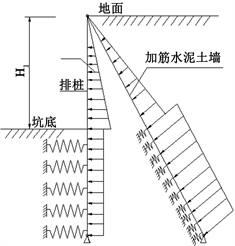Figure 2. Finite element mechanical model of herringbone bracing structure

3.2. 土压力确定

1) 墙后土体作用在墙上的土压力

${\sigma }_{a}=\gamma z{K}_{a}-2c\sqrt{{K}_{a}}$ (1)

${E}_{a}^{,}=\frac{1}{2}\left(\gamma {H}_{1}{K}_{a}-2c\sqrt{{K}_{a}}\right){H}_{1}$ (2)

${E}_{a}=\frac{1}{2\mathrm{sin}\alpha }\left(\gamma {H}_{1}{K}_{a}-2c\sqrt{{K}_{a}}\right){H}_{1}$ (3)

${\sigma }_{a1}+{\sigma }_{b1}=\gamma z{K}_{a}-2c\sqrt{{K}_{a}}$ (4)

${\sigma }_{b2}=\gamma {H}_{1}{K}_{a}-2c\sqrt{{K}_{a}}$ (5)

$\begin{array}{l}{\sigma }_{a1}={K}_{1}\left(\gamma z{K}_{a}-2c\sqrt{{K}_{a}}\right)\\ {\sigma }_{b1}={K}_{2}\left(\gamma z{K}_{a}-2c\sqrt{{K}_{a}}\right)\\ {K}_{1}+{K}_{2}=1\end{array}\right\}$ (6)

$\begin{array}{l}{K}_{1}=\frac{{M}_{E}}{M}=\frac{{b}^{2}}{{H}_{1}^{2}{\mathrm{tan}}^{2}{\varphi }_{D}}\\ {\varphi }_{D}=2\left\{{45}^{\circ }-\text{arc}\left[\mathrm{tan}\left({45}^{\circ }-\frac{\varphi }{2}\right)\right]-\frac{2c}{\gamma {H}_{1}}\right\}\end{array}\right\}$ (7)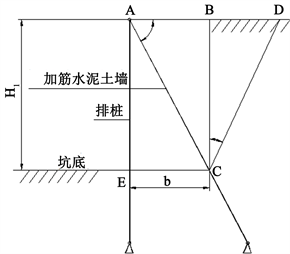Figure 3. Diagram of distribution coefficient calculation

2) 桩墙之间土体作用在排桩上的土压力

3.3. 斜向水泥土墙与土的侧摩擦阻力确定

$\tau \left(z\right)=K\gamma z\mathrm{tan}\phi \left[1-\mathrm{exp}\left(-\frac{ks}{{s}_{u}-s}\right)\right]$ (8)

3.4. 人字形支护结构平面杆系有限元力学模型及计算实现

1) 排桩及水泥土墙的刚度矩阵

${k}_{p}^{e}=\left[\begin{array}{cccc}12EI/{l}^{3}& 6EI/{l}^{2}& -12EI/{l}^{3}& 6EI/{l}^{2}\\ 6EI/{l}^{2}& 4EI/l& -6EI/{l}^{2}& 2EI/l\\ -12EI/{l}^{3}& -6EI/{l}^{2}& 12EI/{l}^{3}& -6EI/{l}^{2}\\ 6EI/{l}^{2}& 2EI/l& -6EI/{l}^{2}& 4EI/l\end{array}\right]$ (9)

${k}_{w}^{e}=\left[\begin{array}{cccccc}\begin{array}{c}EA/l\\ 0\\ 0\\ -EA/l\\ 0\\ 0\end{array}& \begin{array}{c}0\\ 12EI/{l}^{3}\\ 6EI/{l}^{2}\\ 0\\ -12EI/{l}^{3}\\ 6EI/{l}^{2}\end{array}& \begin{array}{c}0\\ 6EI/{l}^{2}\\ 4EI/l\\ 0\\ -6EI/{l}^{2}\\ 2EI/l\end{array}& \begin{array}{c}-EA/l\\ 0\\ 0\\ EA/l\\ 0\\ 0\end{array}& \begin{array}{c}0\\ -12EI/{l}^{3}\\ -6EI/{l}^{2}\\ 0\\ 12EI/{l}^{3}\\ -6EI/{l}^{2}\end{array}& \begin{array}{c}0\\ 6EI/{l}^{2}\\ 2EI/l\\ 0\\ -6EI/{l}^{2}\\ 4EI/l\end{array}\end{array}\right]$ (10)

${\left\{f\right\}}_{i}={\left[{k}_{b}^{e}\right]}_{i}{\left\{w\right\}}_{i}$ (11)

${\left\{w\right\}}_{i}={\left\{\begin{array}{cccc}{w}_{j-1}& {\theta }_{j-1}& {w}_{j}& {\theta }_{j}\end{array}\right\}}_{i}^{\text{T}}$ 为第i单元节点位移列阵；

${\left\{w\right\}}_{i}={\left\{\begin{array}{cccccc}{u}_{j-1}& {w}_{j-1}& {\theta }_{j-1}& {u}_{j}& {w}_{j}& {\theta }_{j}\end{array}\right\}}_{i}^{\text{T}}$ 为第i单元节点位移列阵。 $\left[{k}_{b}^{e}\right]$ 对应排桩或水泥土墙的单元刚度矩阵。

${R}_{j}={K}_{j}{w}_{j}$ (12)

$\left\{F\right\}=\left[{K}_{B}\right]\left\{w\right\}$ (13)

$\left[{K}_{B}\right]$ 对应排桩或水泥土墙的整体刚度矩阵。

2) 地基刚度方程

$\left\{R\right\}=\left[{K}_{S}\right]\left\{s\right\}$ (14)

$\left\{R\right\}={\left\{{R}_{1},{R}_{M1},\cdots ,{R}_{m},{R}_{Mm}\right\}}^{\text{T}},\text{\hspace{0.17em}}\text{\hspace{0.17em}}\left\{s\right\}={\left\{{s}_{1},{\theta }_{1},\cdots ,{s}_{m},{\theta }_{m}\right\}}^{\text{T}}$

$\left\{R\right\}={\left\{{N}_{1},{R}_{1},{R}_{M1},\cdots ,{N}_{m},{R}_{m},{R}_{Mm}\right\}}^{\text{T}},\text{\hspace{0.17em}}\text{\hspace{0.17em}}\left\{s\right\}={\left\{{u}_{1},{s}_{1},{\theta }_{1},\cdots ,{u}_{m},{s}_{m},{\theta }_{m}\right\}}^{\text{T}}$

3) 排桩或斜向加筋水泥土地下连续墙与地基相互作用的整体刚度方程

$\left\{P\right\}=\left[A\right]\left\{w\right\}$ (15)

4) 人字形支护结构整体刚度方程

$\left\{F\right\}=\left[K\right]\left\{\delta \right\}$ (16)

$\begin{array}{l}桩底为铰支时:{M}_{n}=0,{w}_{n}=0\\ 桩底为固支时:{w}_{n}=0,{\phi }_{n}=0\\ 墙底为铰支时:{M}_{n}=0,{u}_{n}={w}_{n}=0\\ 桩底为固支时:{u}_{n}={w}_{n}=0,{\phi }_{n}=0\end{array}\right\}$(17)

4. 工程算例与验证

4.1. 工程概况Table 1. Physical parameters of soil

4.2. 支护结构设计计算

$m=\frac{0.2{\phi }^{2}-\phi +c}{{v}_{b}}$ (18)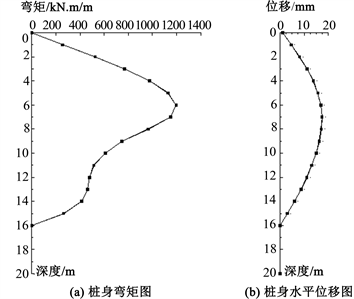Figure 4. Calculation results of row piles of herringbone supporting structure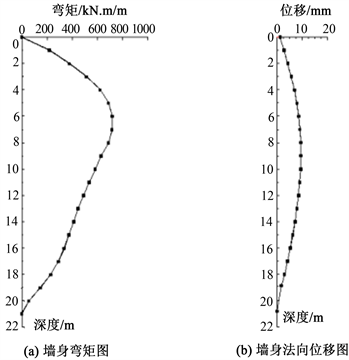Figure 5. Calculation results of cement-soil of herringbone supporting structure

4.3. 工程实测结果分析

5. 结论

 何颐华, 杨斌, 金宝森, 等. 双排护坡桩试验与计算的研究[J]. 建筑结构学报, 1996, l7(2): 58-66.

 戴智敏, 阳凯凯. 深基坑双排桩支护结构体系受力分析与计算[J]. 信阳师范学院学报, 2002(3): 348-351.

 郑刚, 李欣, 刘畅. 考虑桩土相互作用的双排桩分析[J]. 建筑结构学报, 2004, 25(1): 99-106.

 应宏伟, 初振环. 带撑双排桩支护结构性状分析[J]. 岩土工程学报, 2006, 28(S1): 1450-1454.

 平扬, 白世伟, 曹俊坚. 深基双排桩空间协同计算理论及位移反分析[J]. 土木工程学报, 2001, 2(34): 79-84.

 吴才德, 龚迪快, 王洁栋. 门架加锚杆复合结构在超大基坑中的应用[J]. 岩土工程学报, 2006, 28(12): 1781-1785.

 吴刚, 白冰, 聂庆科. 深基坑双排桩支护结构设计计算方法研究[J]. 岩土力学, 2008, 29(10): 2753-2758.

 刘泉声, 付建军. 考虑桩土效应的双排桩模型及参数研究岩土力学, 2011, 32(2): 481-486.

 刘钊. 双排支护结构分析及试验研究[J]. 岩土工程学报, 1992, 14(5): 76-80.

 龚晓南. 土工计算机分析[M]. 北京: 中国建筑工业出版社, 2000: 137.

Top